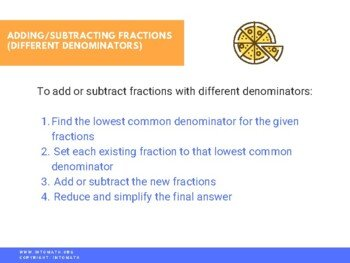CA\$2.00

Adding and subtracting fractions involves representing them as a part of the same whole. Thus, their denominators must be the same. In order to bring them to the same denominator, we need to change all of the existing denominators while at the same time changing the numerator such that the fractions remain equivalent to the original ones.

If two fractions with different denominators are being added or subtracted, first bring them to the Lowest Common Denominator by determining their Least Common Multiple.

For example, for denominators 2 and 5, the Lowest Common Denominator is 10 – the lowest number divisible by both given denominators.